# Audio Preamp Circuit for a Microcontroller

#### Engineer9001

Joined Dec 13, 2019
22
I made a pre-amp audio circuit but it doesn't seem to work. I want to amplify an audio signal so that it has a DC offset of 1.8V.
I tried to simulate a similar circuit in KiCad and the simulation seems to work ok.

Below is the circuit I designed and assembled. On the scope, the voltage is as follows:
Q1 (2N3904)
Base Voltage: ~1V
Collector Voltage: ~400mV
Emitter Voltage: ~380mV

The C9 capacitor has a 0-ohm resistor in place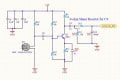Here is the KiCad circuit and simulation: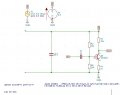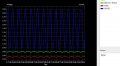Can anybody help me with my circuit?

#### peterdeco

Joined Oct 8, 2019
484
I did this and it worked perfectly. Going by the top schematic remove your R15. Change your R10 to 1 megohm and connect it from base to collector. Change R9 to 10K. Remove R12, R13 and C10. Change your R14 to a lower value. I think I used 470 ohms. Using a PN2222A (similar) I got 1.6V on the collector. I also didn't have your C13 because I was aiming for a voltage gain of about 20. I also used 0.1uF discs for your C11 and C9.

#### Alec_t

Joined Sep 17, 2013
13,319
Remove C9 if you want a DC offset at the mcu input.

#### DickCappels

Joined Aug 21, 2008
9,607
Correct! (There is a note on the schematic that says C9 is to be a 0 Ω resistor.)

•redrooster01

#### Audioguru again

Joined Oct 21, 2019
5,552
Yout voltages are much different to my calculations and to my simulation.
I think your transistor is biased wrongly causing it to be saturated. I fixed it (my resistor numbers are different than yours):

#### Engineer9001

Joined Dec 13, 2019
22
Thanks for all the replies!

Yout voltages are much different to my calculations and to my simulation.
I think your transistor is biased wrongly causing it to be saturated. I fixed it (my resistor numbers are different than yours):
Thanks for the step-by-step circuit analysis. It looks like my circuit is biased incorrectly, however, why does the simulation work out? Maybe my spice parameters for the 2N3904 is incorrect.

So if I use 15k/5k for Rc/Re respectively, I have a gain of only 3?
What is the typical procedure when designing a single amplifier circuit?

Here are my circuit parameters:
- I'm using this MIC as input.
- 3.3V Power
- The amplified signal should swing from 0.2V - 2.9V (DC Offset at 3.3V/2=1.65V)

How do I determine how much gain I need? (dependent on input signal? what is the typical p-p voltage output from a MIC?)
How do I determine how much collector current I need? (maybe dependent on output requirements? uC)

So if I want to design a circuit with the collector voltage at 1.65V and a gain of 10, would this be the procedure:
1) Pick Rc arbitrarily: 5k
2) If gain is 10, then Re= 5k/10 = 500
3) Ic = (3.3V-1.65V) / Rc = 0.33mA = Ie
4) Since Ie = 0.33mA, Ve = 500 * 0.33mA = 0.165V
5) In order to bias properly, Vb = Ve + 0.6 = 0.165V + 0.6 = 0.765V
6) Design the current of R11 so that it is Ie / 10 ? => 0.033mA
7) For I(R11) of 0.033mA, R11 = (3.3V - Vb) / I(R11) => R11 = (3.3V - 0.765V) / 0.033mA = 76,8181 (round up: 80k)
8) Calculate for R15 = Vb / I(R11) = 0.765V / 0.033mA = 23,181 (round down: 22k)

With the values above I couldn't get it to simulate. I reduced R11 and R15 by 10 (8k, 2.2k) to match the current of Ic to I(R11) and it did simulate but not to my design specifications.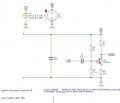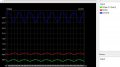#### Alec_t

Joined Sep 17, 2013
13,319
it did simulate but not to my design specifications.
Probably because Vbe is not exactly 0.6V and transistor beta is not 10. [A value of 10 is appropriate if the transistor is being used as a switch, rather than in its linear region].
R11 is not on your post #6 schematic.

#### Audioguru again

Joined Oct 21, 2019
5,552
Your base bias resistor values are Way Too Low. Then they short away a lot of signal from the electret mic and the input coupling capacitor value of only 0.1uF cuts all low audio frequencies.
With your 5k (also a low value) for the collector resistor the collector current is about 1V/5k= 0.2mA. Then the 2N3904 has a typical hFE of about 100 for its low collector-emitter voltage. Then its typical base current is 0.2mA/100= 2uA and the current in the base bias voltage divider should be 2uA x 10= 20uA then their total resistance is 3.3V/20uA= 165k. The total can be as low as about 51k then the input coupling capacitor value of 0.1uF can pass frequencies down to about 100Hz.

Your 8k (not a standard value) and 2.2k voltage divider causes the base to be at 0.71V then the emitter is at 0.11V and the emitter current is 0.11V/500=0.22mA. Then the collector voltage is 3.3V - (0.22mA x 5k)= 2.2V (too high) and can swing up to 3.3V and down to 0.2V for a total p-p output of 3.1V (into an impedance of 20k or higher). Only 2.2V p-p can be symmetrical.

You wrongly said the gain was only 3 but you forgot that the emitter capacitor was a short circuit to AC. My previous simulation shows a distorted gain of about 58 times at 1kHz. A mic output is about 10mV RMS for a conversation level 10cm away from your mouth. Then to have an output of 1V you need a gain of 100 times but more is needed if the person at the mic has a faint voice level.

I think your simulation program has the Covid-19 virus, mine works fine with your 80k and 22k base bias resistors but then the
collector voltage is too high and causes clipping as shown here:

#### Attachments

•redrooster01

#### Engineer9001

Joined Dec 13, 2019
22
Probably because Vbe is not exactly 0.6V and transistor beta is not 10. [A value of 10 is appropriate if the transistor is being used as a switch, rather than in its linear region].
R11 is not on your post #6 schematic.
Sorry I was referring to the very first schematic I had (altium) instead of the KiCad schematic for simulation. I'll update the KiCad schmatic designators to reflect the one in the altium schematic

I see. I mixed up the base current with the biasing current. Base current is Emitter current divided by 100. And biasing current is base current times 10.

Your base bias resistor values are Way Too Low. Then they short away a lot of signal from the electret mic and the input coupling capacitor value of only 0.1uF cuts all low audio frequencies.
With your 5k (also a low value) for the collector resistor the collector current is about 1V/5k= 0.2mA. Then the 2N3904 has a typical hFE of about 100 for its low collector-emitter voltage. Then its typical base current is 0.2mA/100= 2uA and the current in the base bias voltage divider should be 2uA x 10= 20uA then their total resistance is 3.3V/20uA= 165k. The total can be as low as about 51k then the input coupling capacitor value of 0.1uF can pass frequencies down to about 100Hz.

Your 8k (not a standard value) and 2.2k voltage divider causes the base to be at 0.71V then the emitter is at 0.11V and the emitter current is 0.11V/500=0.22mA. Then the collector voltage is 3.3V - (0.22mA x 5k)= 2.2V (too high) and can swing up to 3.3V and down to 0.2V for a total p-p output of 3.1V (into an impedance of 20k or higher). Only 2.2V p-p can be symmetrical.

You wrongly said the gain was only 3 but you forgot that the emitter capacitor was a short circuit to AC. My previous simulation shows a distorted gain of about 58 times at 1kHz. A mic output is about 10mV RMS for a conversation level 10cm away from your mouth. Then to have an output of 1V you need a gain of 100 times but more is needed if the person at the mic has a faint voice level.

I think your simulation program has the Covid-19 virus, mine works fine with your 80k and 22k base bias resistors but then the
collector voltage is too high and causes clipping as shown here:
What would be the typical collector current value range for an audio preamp? This would dictate what resistor values to choose for the Collector and Emitter (R9, R14)

I do have the C13 emitter capacitor in the schematic, but it's Not Fitted on the actual PCB. What would be the pros/cons using this capacitor?

What would be the maximum amount of gain that could be designed without distortion using a single transistor amplifier?

I'm using KiCad v5.1.5. Not sure why it's acting funny. The spice model parameters for 2N3904 is:
Code:
.model 2N3904 NPN(IS=1E-14 VAF=100
+  Bf=300 IKF=0.4 XTB=1.5 BR=4
+  CJC=4E-12  CJE=8E-12 RB=20 RC=0.1 RE=0.1
+  TR=250E-9  TF=350E-12 ITF=1 VTF=2 XTF=3 Vceo=40 Icrating=200m mfg=Philips)
I simulated using the same values in your latest post, but it seems the collector current is at 3V. Not sure why that is.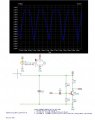I've redone the calculations and have calculated 1.82V at the collector by changing only the R11 resistor to 75k.

-> Design Vc = 1.6V
1) Voltage drop across R9 (Collector resistor) should be: Vr9 = 3.3V - Vc = 3.3V - 1.6V = 1.7V
2) If using 5k ohm resistor for R9, the Collector current is => 1.7V / 5000 = 0.34mA
3) If using a 500 ohm resistor for R14 (Emitter resistor), the voltage at the Emitter (Ve) is: 500 x 0.34mA = 0.17V
4) Voltage at the Base is: Vb = Ve + 0.6 = 0.77V
5) Base current is the Emitter/Collector current divided by 100: Ib = Ie / 100 = 3.4uA
6) The Biasing current is equal to: Ib x 10 = 34uA
7) R15 = Vb / Ibias = 0.77V / 34uA = 22,647 (22k-ohm)
8) R11 = (3.3V - 0.77V) / 34uA = 74,411 (75k-ohm)

9) Actual Vb using standard resistors of 22k, 75k comes out to: ( 22k / (22k + 75k) ) * 3.3V) = 0.748V
10) Ve = Vb - 0.6 = 0.148V
11) Ie = Ve / 500 = 0.296mA
12) Vc = 3.3V - (0.296mA x 5000) = 1.82V

The collector voltage is not exactly the designed 1.6V because of the limitation of using standard resistor values.

However, using 75k for R11 still gives me a simulation of the Collector voltage at around 3V. I think the KiCad simulation parameters must be off.

#### Alec_t

Joined Sep 17, 2013
13,319
You have assumed a transistor beta of 100 in your calculations, but the simulation model has a beta of 300.

#### Engineer9001

Joined Dec 13, 2019
22
You have assumed a transistor beta of 100 in your calculations, but the simulation model has a beta of 300.
I changed the Bf value from 300 to 100, but the simulation is about the same.

How do I configure the correct spice model parameters for the 2N3904? Where can I find reference documentation on what the meaning of each parameter is? (IKF, XTB, BR, CJE...etc)

#### Alec_t

Joined Sep 17, 2013
13,319

#### Audioguru again

Joined Oct 21, 2019
5,552
Your simulation shows an input level of 120mV peak! If you scream as loud as you can with the mic touching your lips then you might get an signal so high.
When you buy a 2N3904 transistor then you get a beta with whatever they have, it might be 50 or might be 300. You must select an emitter resistor value and power supply voltage that allows the transistor to work properly within that range.
Your supply voltage is Way Too Low but the collector current is fine. The supply voltage should be much higher to allow for loud sound levels sometimes and so that the transistor's biasing changes caused by beta, Vbe and temperature changes allow the transistor to work with low distortion. Then attenuate the output (or use a volume control) to feed your ADC.
A single transistor produces distortion as I showed in my simulations. The higher is the gain then the higher is the distortion. An emitter capacitor completely kills negative feedback but allows high gain. Before audio opamps were used, hifi preamps used two transistors with plenty of negative feedback and gain. With a higher supply voltage then the collector will not swing close to cutoff where distortion (top squashing) is the worst. I am talking about signal levels lower than clipping.

Don't rely on one simulation unless you are doing school work and making only one project where you can pick parts that match the beta your transistor has. Make a simulation of the lowest then of the highest beta you expect and also consider Vbe differences of transistors and temperature changes.

#### MrChips

Joined Oct 2, 2009
28,106

#### Audioguru again

Joined Oct 21, 2019
5,552
Your sinewave looks good with this very high input level of 50mV peak. The preamp gain is much too low for normal levels.
The low value of the input coupling capacitor reduces 114Hz -3dB and cuts lower frequencies at -6dB per octave.

EDIT: The DC on your circuit is dropping with time on the waveform because you start the simulation immediately before the input coupling capacitor is charged in about 50ms. I always begin my audio simulations with a 100ms to 200ms delay.

#### Engineer9001

Joined Dec 13, 2019
22

#### Engineer9001

Joined Dec 13, 2019
22
Your simulation shows an input level of 120mV peak! If you scream as loud as you can with the mic touching your lips then you might get an signal so high.
When you buy a 2N3904 transistor then you get a beta with whatever they have, it might be 50 or might be 300. You must select an emitter resistor value and power supply voltage that allows the transistor to work properly within that range.
Your supply voltage is Way Too Low but the collector current is fine. The supply voltage should be much higher to allow for loud sound levels sometimes and so that the transistor's biasing changes caused by beta, Vbe and temperature changes allow the transistor to work with low distortion. Then attenuate the output (or use a volume control) to feed your ADC.
A single transistor produces distortion as I showed in my simulations. The higher is the gain then the higher is the distortion. An emitter capacitor completely kills negative feedback but allows high gain. Before audio opamps were used, hifi preamps used two transistors with plenty of negative feedback and gain. With a higher supply voltage then the collector will not swing close to cutoff where distortion (top squashing) is the worst. I am talking about signal levels lower than clipping.

Don't rely on one simulation unless you are doing school work and making only one project where you can pick parts that match the beta your transistor has. Make a simulation of the lowest then of the highest beta you expect and also consider Vbe differences of transistors and temperature changes.
Thanks!
In a scenario where you only have 3.3V power. Would you recommend using a boost converter to up the voltage in order to get better gains?
This is just a personal project I'm working on. It's really just meant to be a sound sensor, but also a study on transistor amplifier circuits.

#### Engineer9001

Joined Dec 13, 2019
22

#### Audioguru again

Joined Oct 21, 2019
5,552
In a scenario where you only have 3.3V power. Would you recommend using a boost converter to up the voltage in order to get better gains?
This is just a personal project I'm working on. It's really just meant to be a sound sensor, but also a study on transistor amplifier circuits.
You can get as much gain as you want with ANY supply voltage. But the circuit has only a single transistor so if the gain is high then the distortion will also be high.
You need a higher supply voltage so that loud voices or music will play loudly without distortion.

You never said what "sounds" you are sensing. Maybe nearby gun shots?? Then distortion does not matter.